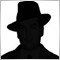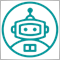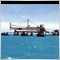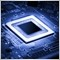• Overview
• Reviews (6)

# ImpBB

The standard Bollinger Bands indicator is calculated only on the basis of 7 price constants and a simple Moving Average (SMA). This modification allows calculating the indicator on the basis of any combination of 4 basic prices: Close, Open, High and Low.

An additional convenience of the indicator is the possibility to select one of the six methods of averaging: simple (SMA), exponential (EMA), smoothed (SMMA), linear weighed (LWMA), double exponential (DEMA), triple exponential (TEMA).

Input parameters:

• Period - the averaging period for calculation of the indicator;
• Shift - shift of the indicator relative to the price chart;
• Deviation - a standard deviation;
• Moving Average mode - the method of averaging: simple (Simple, SMA), exponential (Exponential, EMA), smoothed (Smoothed, SMMA), linear weighed (Linear Weighted, LWMA), double exponential (Double Exponential, DEMA) or triple exponential Moving Average (Triple Exponential, TEMA);
• Close - weight coefficient of CLOSE price for calculation of the individual price;
• Open - weight coefficient of OPEN price for calculation of the individual price;
• High - weight coefficient of HIGH price for calculation of the individual price;
• Low - weight coefficient of LOW price for calculation of the individual price.

The example 1. Calculate the indicator by OPEN price: Close=0, Open=1, High=0, Low=0.
The example 2. Calculate the indicator by WEIGHTED price: Close=2, Open=0, High=1, Low=1.
The example 3. Calculate the indicator by individual price constant: Close=6, Open=7, High=1, Low=3. Price=(6*Close + 7*Open + 1*High + 3*Low)/17.16100
Nikolay Gaylis 2019.01.14 08:15

User didn't leave any comment to the rating12010
2018.02.04 20:56

User didn't leave any comment to the rating5698
2017.11.09 14:47

User didn't leave any comment to the rating6155
2017.07.21 17:34

User didn't leave any comment to the rating7170
2016.03.12 22:12

User didn't leave any comment to the rating7827
2014.04.08 12:34

User didn't leave any comment to the rating